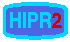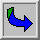# Sobel Edge DetectorCommon Names: Sobel, also related is Prewitt Gradient Edge Detector

## Brief Description

TheSobel operator performs a 2-D spatial gradient measurement on an image and so emphasizes regions of high spatial frequency that correspond to edges. Typically it is used to find the approximate absolute gradient magnitude at each point in an input grayscale image.

## How It Works

In theory at least, the operator consists of a pair of 3×3 convolution kernels as shown in Figure 1. One kernel is simply the other rotated by 90°. This is very similar to the Roberts Cross operator.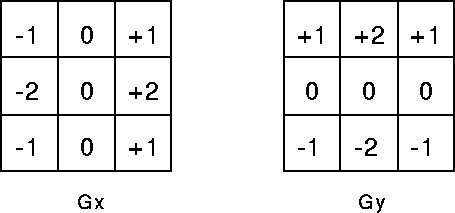Figure 1 Sobel convolution kernels

These kernels are designed to respond maximally to edges running vertically and horizontally relative to the pixel grid, one kernel for each of the two perpendicular orientations. The kernels can be applied separately to the input image, to produce separate measurements of the gradient component in each orientation (call these Gx and Gy). These can then be combined together to find the absolute magnitude of the gradient at each point and the orientation of that gradient. The gradient magnitude is given by: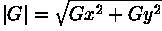Typically, an approximate magnitude is computed using: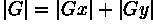which is much faster to compute.

The angle of orientation of the edge (relative to the pixel grid) giving rise to the spatial gradient is given by: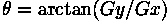In this case, orientation 0 is taken to mean that the direction of maximum contrast from black to white runs from left to right on the image, and other angles are measured anti-clockwise from this.

Often, this absolute magnitude is the only output the user sees --- the two components of the gradient are conveniently computed and added in a single pass over the input image using the pseudo-convolution operator shown in Figure 2.Figure 2 Pseudo-convolution kernels used to quickly compute approximate gradient magnitude

Using this kernel the approximate magnitude is given by:## Guidelines for Use

The Sobel operator is slower to compute than the Roberts Cross operator, but its larger convolution kernel smooths the input image to a greater extent and so makes the operator less sensitive to noise. The operator also generally produces considerably higher output values for similar edges, compared with the Roberts Cross.

As with the Roberts Cross operator, output values from the operator can easily overflow the maximum allowed pixel value for image types that only support smallish integer pixel values (e.g. 8-bit integer images). When this happens the standard practice is to simply set overflowing output pixels to the maximum allowed value. The problem can be avoided by using an image type that supports pixel values with a larger range.

Natural edges in images often lead to lines in the output image that are several pixels wide due to the smoothing effect of the Sobel operator. Some thinning may be desirable to counter this. Failing that, some sort of hysteresis ridge tracking could be used as in the Canny operator.

The imageshows the results of applying the Sobel operator toCompare this with the equivalent Roberts Cross output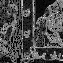Note that the spurious noise that afflicted the Roberts Cross output image is still present in this image, but its intensity relative to the genuine lines has been reduced, and so there is a good chance that we can get rid of this entirely by thresholding. Also, notice that the lines corresponding to edges have become thicker compared with the Roberts Cross output due to the increased smoothing of the Sobel operator.

The image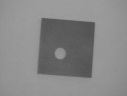shows a simpler scene containing just a single flat dark object against a lighter background. Applying the Sobel operator produces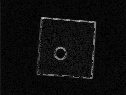Note that the lighting has been carefully set up to ensure that the edges of the object are nice and sharp and free of shadows.

The Sobel edge detector can also be applied torange images likeThe corresponding edge image is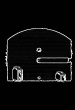All edges in the image have been detected and can be nicely separated from the background using a threshold of 150, as can be seen inAlthough the Sobel operator is not as sensitive to noise as the Roberts Cross operator, it still amplifies high frequencies. The image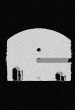is the result of adding Gaussian noise with a standard deviation of 15 to the original image. Applying the Sobel operator yields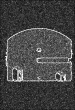and thresholding the result at a value of 150 produces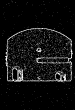We can see that the noise has increased during the edge detection and it is no longer possible to find a threshold which removes all noise pixels and at the same time retains the edges of the objects.

The object in the previous example contains sharp edges and its surface is rather smooth. Therefore, we could (in the noise-free case) easily detect the boundary of the object without getting any erroneous pixels. A more difficult task is to detect the boundary ofbecause it contains many fine depth variations (i.e. resulting in intensity changes in the image) on its surface. Applying the Sobel operator straightforwardly yieldsWe can see that the intensity of many pixels on the surface is as high as along the actual edges. One reason is that the output of many edge pixels is greater than the maximum pixel value and therefore they are `cut off' at 255. To avoid this overflow we scale the range image by a factor 0.25 prior to the edge detection and then normalize the output, as can be seen in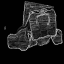Although the result has improved significantly, we still cannot find a threshold so that a closed line along the boundary remains and all the noise disappears. Compare this image with the results obtained with the Canny edge detector.## Common Variants

A related operator is the Prewitt gradient edge detector (not to be confused with the Prewitt compass edge detector). This works in a very similar way to the Sobel operator but uses slightly different kernels, as shown in Figure 3. This kernel produces similar results to the Sobel, but is not as isotropic in its response.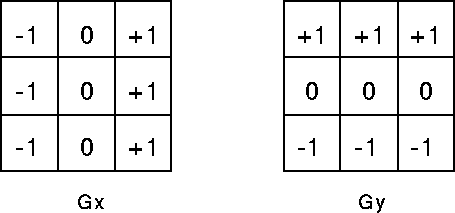## Interactive Experimentation

You can interactively experiment with this operator by clicking here.

## Exercises

1. Experiment with thresholding the example images to see if noise can be eliminated while still retaining the important edges.

2. How does the Sobel operator compare with the Roberts Cross operator in terms of noise rejection, edge detection and speed?

3. How well are the edges located using the Sobel operator? Why is this?

4. Apply the Sobel operator to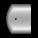and see if you can use thresholding to detect the edges of the object without also selecting noisy pixels. Compare the results with those obtained with the Roberts Cross operator.

5. Under what conditions would you want to use the Sobel rather than the Roberts Cross operator? And when would you not want to use it?

## References

R. Gonzalez and R. Woods Digital Image Processing, Addison Wesley, 1992, pp 414 - 428.

R. Boyle and R. Thomas Computer Vision: A First Course, Blackwell Scientific Publications, 1988, pp 48 - 50.

E. Davies Machine Vision: Theory, Algorithms and Practicalities, Academic Press, 1990, Chap. 5.

D. Vernon Machine Vision, Prentice-Hall, 1991, Chap. 5.

## Local Information